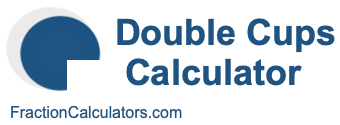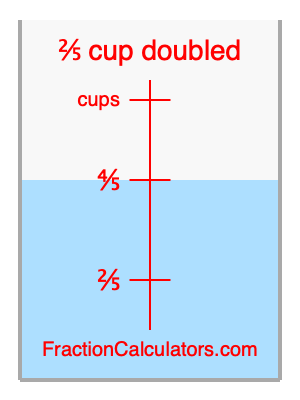What is 2/5 cup doubled?Here we will calculate 2/5 cup doubled and illustrate 2/5 cup doubled on a measuring cup. What is 2/5 cup doubled? 2/5 cup doubled is twice 2/5 cup. Therefore, to double 2/5 cup, we multiply 2/5 by 2. Here is the formula, the math, and the answer to 2/5 cup doubled:

Cup × 2 = Cup doubled
2/5 × 2 = 4/5
2/5 cup doubled = 4/5 cup

Below is an illustration of a measuring cup. We show 2/5 cup and 2/5 cup doubled (4/5 cup) so you can see where they are in relation to each other.As you can see, we filled up our measuring cup to 2/5 cup doubled. In other words, we filled it up to 4/5, which is two times 2/5.

Double Cups Calculator
Here you can double another cup measurement.

What is cups doubled?

What is 1/2 doubled?
Here is the next measurement on our list that we have doubled for you.